﻿ 基于卷积特征和贝叶斯分类器的人脸识别
«上一篇文章快速检索 高级检索

 智能系统学报2018, Vol. 13Issue (5): 769-775  DOI: 10.11992/tis.2017060520

### 引用本文FENG Xiaorong, HUI Kanghua, LIU Zhendong. Face recognition based on convolution feature and Bayes classifier[J]. CAAI Transactions on Intelligent Systems, 2018, 13(5): 769-775. DOI: 10.11992/tis.201706052.### 文章历史

Face recognition based on convolution feature and Bayes classifier
FENG Xiaorong, HUI Kanghua, LIU Zhendong
School of Computer Science and Technology, Civil Aviation University of China, Tianjin 300300, China
Abstract: To solve the difficulty of feature extraction of the traditional face recognition algorithm, a new method based on convolution feature and Bayes classifier is proposed, which uses convolution neural network to extract facial features and principal component analysis (PCA) to reduce the feature dimension, and finally, employs a Bayes classifier to classify the features. Experiments were carried out on the ORL face database, and a recognition accuracy of 99% was achieved. The experimental results show that the face features extracted by the convolution neural network have a strong degree of recognition. Therefore, the accuracy of face recognition in feature extraction can be effectively improved by combining PCA and Bayes classifier with convolution neural network.
Key words: face recognition    convolutional neural network    pattern recognition    deep learning    Bayes classifier

1 相关工作 1.1 卷积神经网络

1.1.1 卷积层

 $x_j^l = f(\sum\limits_{i \in M_{j}} {x_i^{l - 1}k_{ij}^l} + b_j^l)$ (1)

1.1.2 亚采样层

 $x_j^l = f(\beta _j^l{\rm{down}}(x_j^{l - 1}) + b_j^l)$ (2)

1.2 PCA算法

PCA即主成分分析法，是一种基于统计思想的降维方法。它通过正交变换，将与其分量相关的原随机向量转化为与其分量不相关的新随机向量，达到降维的目的。

$p$ 维的随机向量 ${{x}} = {[{x_1}\;{x_2} \cdots {x_p}]^{\rm{T}}}$ $N$ 个训练样本 ${{{x}}_i} = {[{x_{i1}}\;{x_{i2}} \cdots {x_{ip}}]^{\rm{T}}},i = 1,2 \cdots ,N$ $N > p$ ，则训练样本的均值 $u$ 可由式(3)计算出来。

 $u = \frac{1}{N}\sum\limits_{i = 1}^N {{x_i}}$ (3)

 ${{Z}} = [{x_1} - u\;{x_2} - u \cdots {x_n} - u]$ (4)

 ${{C}} = \frac{{{{Z}} \cdot {{{Z}}^{\rm{T}}}}}{N}$ (5)

 $\alpha = \frac{{\displaystyle\sum\limits_{i = 1}^k {{\lambda _i}} }}{{\displaystyle\sum\limits_{i = 1}^p {{\lambda _i}} }}$ (6)

 ${{Q}} = {{{U}}^{\rm{T}}}{{Z}}$ (7)

${{Q}}$ 即为降维之后的数据。

1.3 朴素贝叶斯分类器

 $P({y_k}|x) = {\rm{max}}\{ P({y_1}|x), P({y_2}|x), \cdots ,P({y_m}|x)\}$ (8)

${y_k}$ 即为贝叶斯分类器判别的未知样本 $x$ 的类别。

1) 构造已知类别的训练样本集合；

2) 统计训练集中各个特征属性在每个类别中的条件概率估计，即计算式(9)所示概率：

 $P({x_1}|{y_1}),P({x_2}|{y_1}), \cdots P({x_n}|{y_1})$ (9)

3) 假定各个特征属性是独立的，根据贝叶斯定理,可得条件概率计算表达式，即

 $P({y_i}|x) = P(x|{y_i})P({y_i})/P(x)$ (10)

4) $P(x)$ 对所有类别都是一样的，因此只要将分子最大化即可，简化后的条件概率表达式为

 $P(x|{y_i})P({y_i}) = P({y_i})\prod\limits_{j = 1}^n {P({x_j}|{y_i})}$ (11)
2 算法详细设计 2.1 算法框架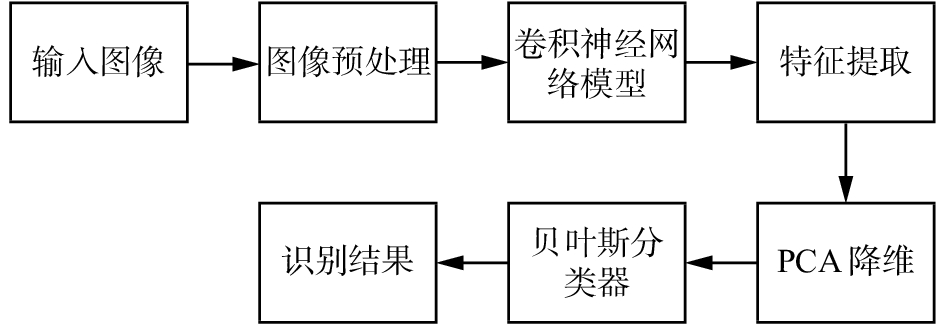Download: 图 1 算法框架 Fig. 1 Schematic diagram of algorithm frame

2.2 数据预处理

1) 调整图像尺寸。通过imresize函数对训练集和测试集中每一幅图像进行缩放处理，由原始的112×92变为28×28。

2) 归一化图像。ORL数据集中的图像为灰度图像，其颜色灰度值一般在0~255，将缩放后图像的原始像素值除以255归一化到[0, 1]。

 ${{x}}' = {{x}}/255$ (12)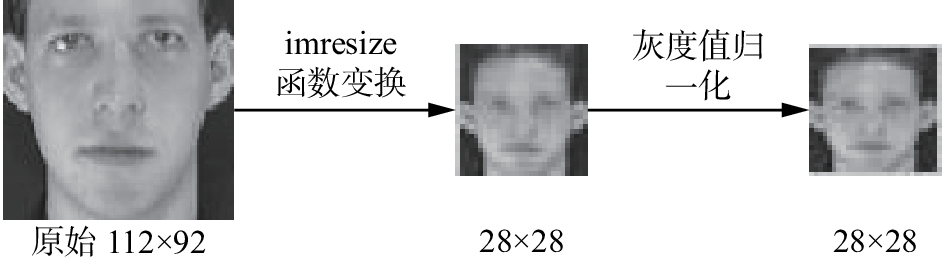Download: 图 2 数据预处理过程 Fig. 2 Data preprocessing
2.3 卷积神经网络结构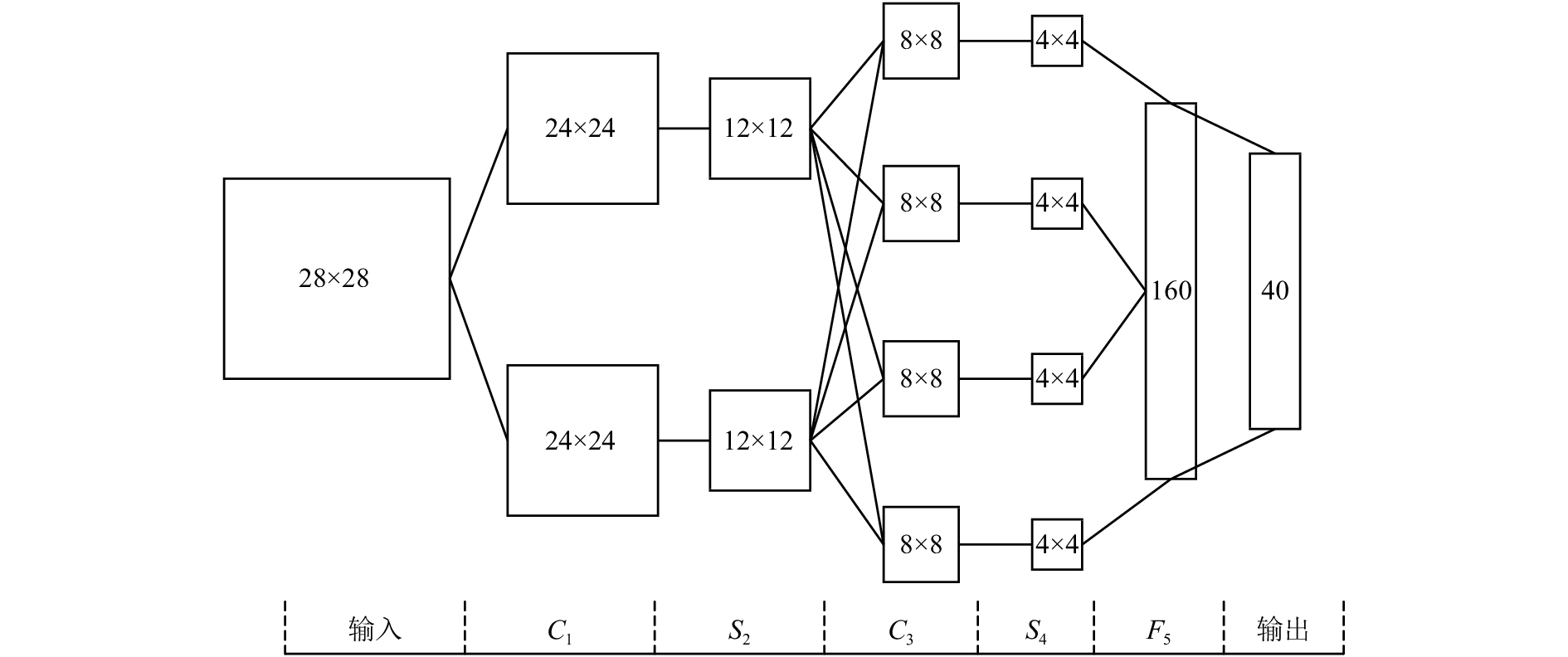Download: 图 3 卷积神经网络层次结构 Fig. 3 Hierarchical structure of convolution neural network

1) 输入层为28×28的灰度图像；

2) 卷积层C1通过6个5×5的卷积核对输入层的灰度图像进行卷积操作，得到6个24×24的特征图；

3) 亚采样层S2在2×2的区域内对C1层的特征图执行均值亚采样，得到6个12×12的特征图；

4) 卷积层C3通过10个5×5的卷积核对S2层的特征图进行卷积操作，得到10个8×8的特征图；

5) 亚采样层S4在2×2的区域内对C3层的特征图执行均值亚采样，得到10个4×4的特征图。此时将S4层的所有特征图连接为一个160维的特征向量；

6) 学习率为1.7，批处理块大小为2，网络阈值函数为sigmoid。

3 实验结果

ORL人脸数据集中部分人脸图像如图4所示。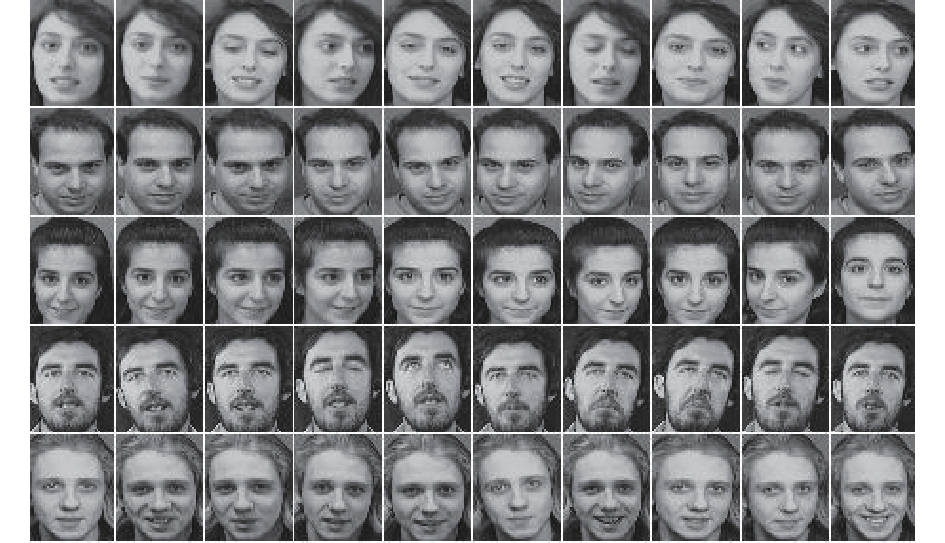Download: 图 4 ORL数据集中部分人脸图像 Fig. 4 Partial face images of ORL dataset

ORL数据集中包含40个不同人物的脸部图像，每个人物包含10张图像，总共400张图像，每个图像原始大小均为112像素×92像素。每个类别中的脸部图像在不同的时间拍摄得到，主要存在光线、面部表情和面部细节上的一些差异。本文随机选取每一个类别图像中的8张作为训练样本，剩下的2张图像作为测试样本，训练集与测试集的比例为4∶1。

3.1 网络结构设计 3.1.1 卷积核尺寸选择

 ${s} = {n} + 1 - {m}$ (13)

ORL人脸图像经2.2节预处理后大小变为28×28，为确保卷积后的特征图大小为整数，将C1C3层卷积核大小设置为5×5。

3.1.2 C3层卷积核个数选择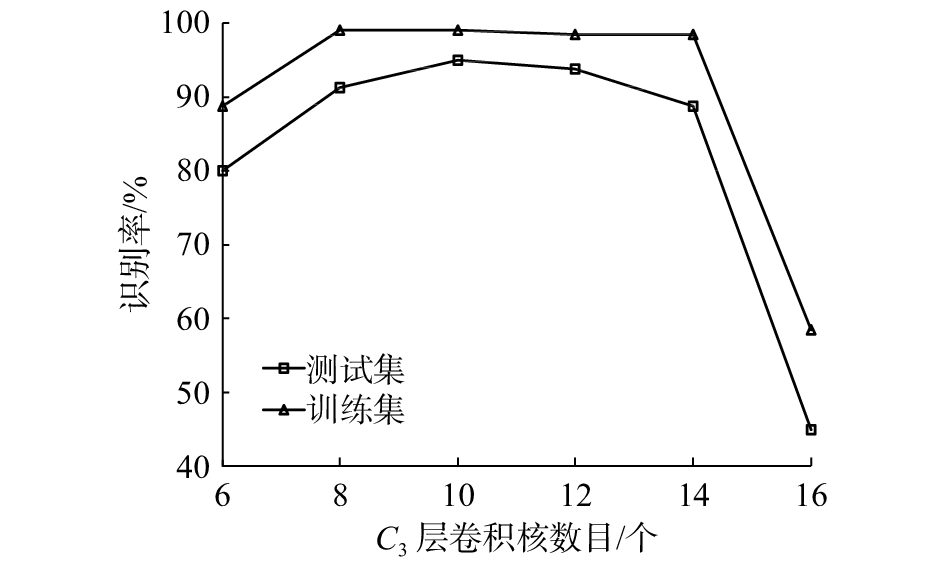Download: 图 5 C3层卷积核个数与识别率关系 Fig. 5 Relationship between the number of C3 layer convolution kernel and recognition accuracy

3.1.3 C1层卷积核个数选择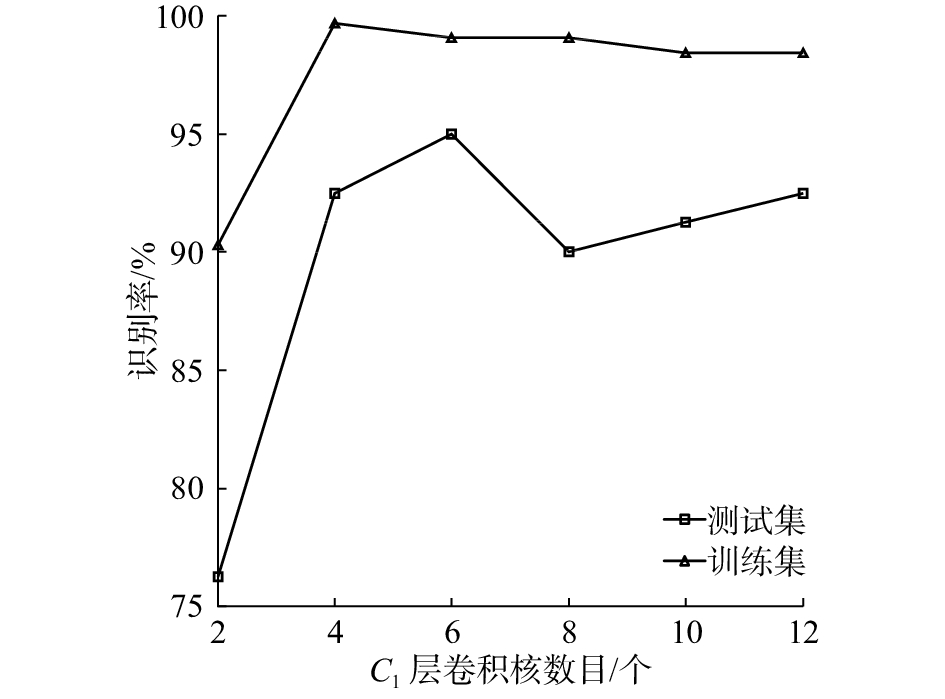Download: 图 6 C1层卷积核个数与识别率关系 Fig. 6 Relationship between the number of C1 layer convolution kernel and recognition accuracy

3.1.4 学习率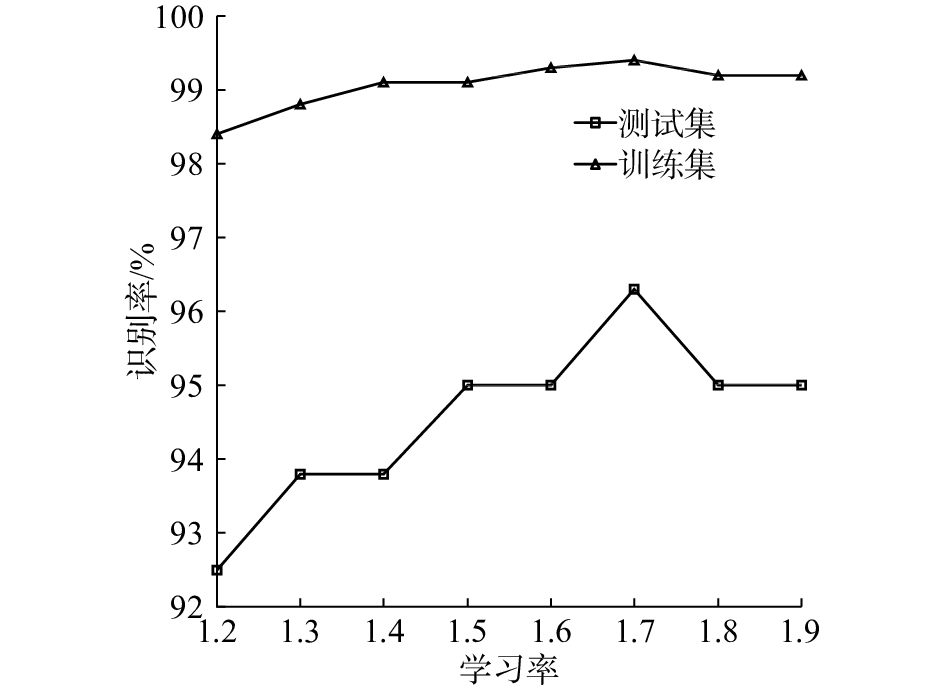Download: 图 7 学习率与识别率关系曲线 Fig. 7 Relationship curve between learning rate and recognition rate

3.2 卷积激活特征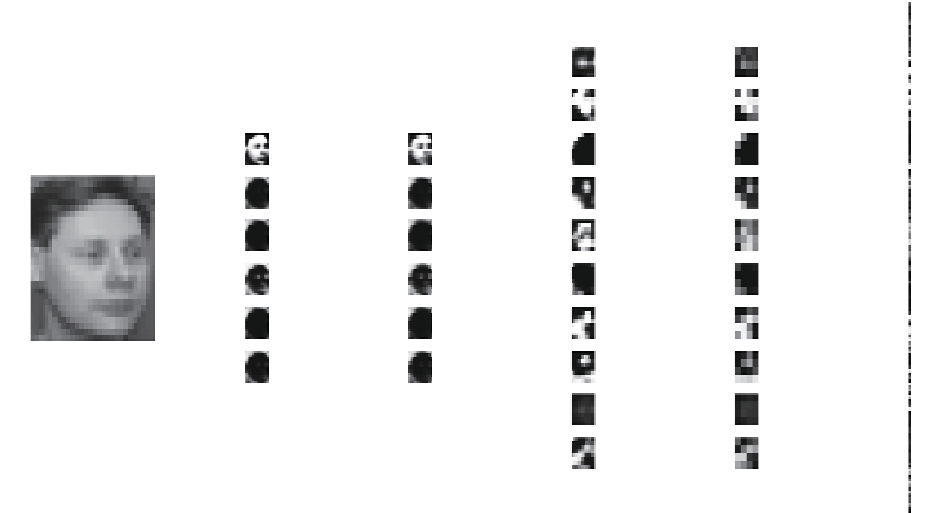Download: 图 8 人脸图像卷积特征 Fig. 8 Convolution feature of face image

3.3 卷积神经网络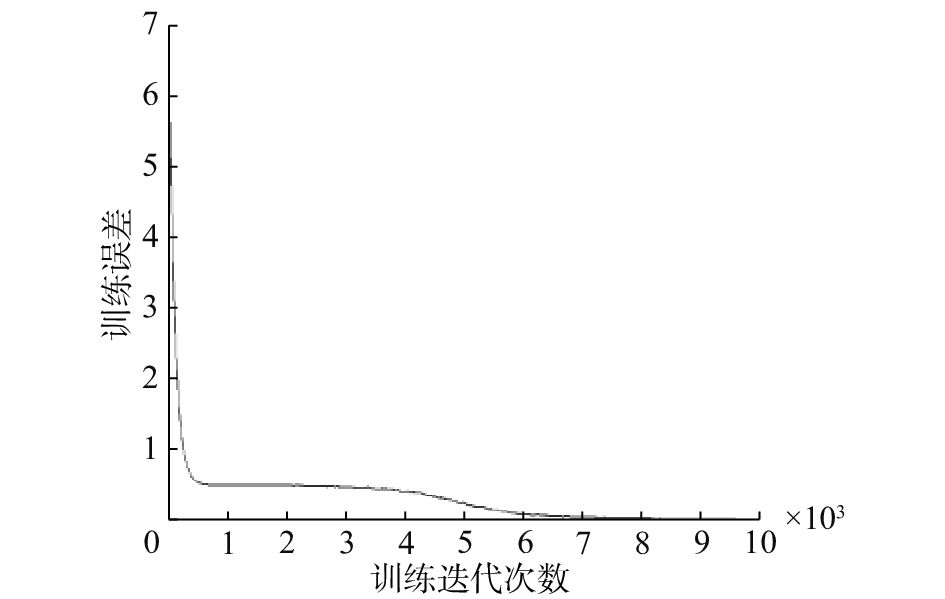Download: 图 9 卷积神经网络训练误差曲线 Fig. 9 Training error curve of convolution neural network

3.4 PCA降维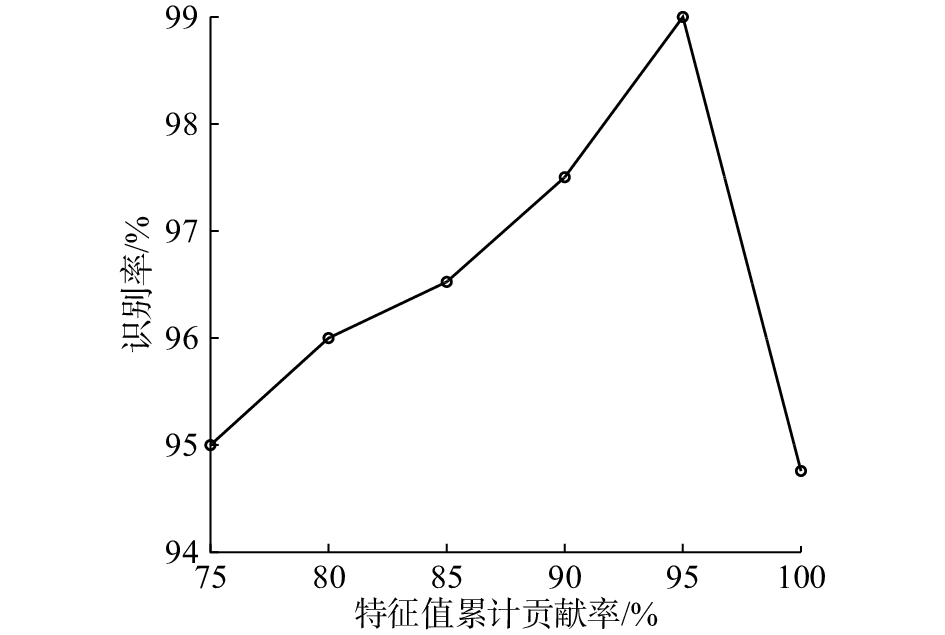Download: 图 10 特征值累积贡献率与识别准确率的关系 Fig. 10 Relationship between eigenvalue cumulative contribution rate and recognition accuracy rate

$\alpha$ 表示特征值累积贡献率，从图10可知，当 $\alpha$ 的取值从100%减少到95%过程中，识别准确率逐渐增加，当 $\alpha =$ 95%时，识别准确率最高，当 $\alpha$ 取值继续减小时，识别准确率逐渐开始下降。这主要是因为，当 $\alpha$ 的取值从100%减少到95%过程中，卷积特征经PCA变换时，冗余信息被去除的越来越多，当 $\alpha$ 取值为95%时，冗余信息几乎被全部剔除，获得的识别准确率也最高；当 $\alpha$ 继续减少时，卷积特征中的部分有用信息也被剔除，导致识别效果越来越差。因此，在利用PCA进行降维压缩时，主成分的维数选择至关重要。在本文人脸识别实验中，选取 $\alpha =$ 95%时的主成分维数，那么经PCA变换之后，原来160维的卷积特征就变为41维的特征，降维效果比较显著。

3.5 对比实验表 1 对比实验结果 Tab.1 Comparison of experimental results

3.6 结果分析

4 结束语

  何春. 人脸识别技术综述[J]. 智能计算机与应用, 2016, 6(5): 112-114. HE Chun. Survey of face recognition technology[J]. Intelligent computers and applications, 2016, 6(5): 112-114. DOI:10.3969/j.issn.2095-2163.2016.05.034 (0)  顼聪, 陶永鹏. 基于改进的LBP人脸识别算法的研究[J]. 微型机与应用, 2016, 35(23): 18-20, 27. XU Cong, TAO Yongpeng. Research of face recognition algorithm based on improved LBP[J]. Microcomputer & its applications, 2016, 35(23): 18-20, 27. (0)  李春利, 沈鲁娟. 基于改进LBP算子的纹理图像分类方法[J]. 计算机工程与设计, 2016, 37(1): 232-236. LI Chunli, SHEN Lujuan. Texture image classification method based on improved LBP operator[J]. Computer engineering and design, 2016, 37(1): 232-236. (0)  SURHONE L M, TENNOE M T, HENSSONOW S F, et al. Histogram of oriented gradients[J]. Betascript publishing, 2016, 12(4): 1368-1371. (0)  ZHANG Chunjie, LIU Jing, LIANG Chao, et al. Image classification using Harr-like transformation of local features with coding residuals[J]. Signal processing, 2013, 93(8): 2111-2118. DOI:10.1016/j.sigpro.2012.09.007 (0)  KRIZHEVSKY A, SUTSKEVER I, HINTON G. ImageNet classification with deep convolutional neural networks[C]//Proceedings of the 25th International Conference on Neural Information Processing Systems. Lake Tahoe, Nevada, 2012: 1097–1105. (0)  YU K, SALZMANN M. Second-order convolutional neural networks[J]. Clinical immunology & immunopathology, 2017, 66(3): 230-238. (0)  LECUN Y, BENGIO Y, HINTON G. Deep learning[J]. Nature, 2015, 521(7553): 436-444. DOI:10.1038/nature14539 (0)  张丹丹, 李雷. 基于PCANet-RF的人脸检测系统[J]. 计算机技术与发展, 2016, 26(2): 31-34. ZHANG Dandan, LI Lei. Face detection system based on PCANet-RF[J]. Computer technology and development, 2016, 26(2): 31-34. (0)  林江豪, 阳爱民, 周咏梅, 等. 一种基于朴素贝叶斯的微博情感分类[J]. 计算机工程与科学, 2012, 34(9): 160-165. LIN Jianghao, YANG Aimin, ZHOU Yongmei, et al. Classification of microblog sentiment based on Naïve Bayesian[J]. Computer engineering and science, 2012, 34(9): 160-165. (0)  许可. 卷积神经网络在图像识别上的应用的研究[D]. 杭州: 浙江大学, 2012. XU Ke. Study of convolutional neural network applied on image recognition[D]. Hangzhou: Zhejiang University, 2012. (0)  陈耀丹, 王连明. 基于卷积神经网络的人脸识别方法[J]. 东北师大学报: 自然科学版, 2016, 48(2): 70-76. CHEN Yaodan, WANG Lianming. Convolutional neural network for face recognition[J]. Journal of Northeast Normal University: Natural Science Edition, 2016, 48(2): 70-76. (0)  BEN Xianye, MENG Weixiao, WANG Kejun, et al. An adaptive neural networks formulation for the two-dimensional principal component analysis[J]. Neural computing and applications, 2016, 27(5): 1245-1261. DOI:10.1007/s00521-015-1922-z (0)  柳振东. 卷积神经网络在图像分类中的研究与应用[D]. 天津: 中国民航大学, 2017. LIU Zhendong. Research and application of convolution neural network in image classification[D]. Tianjin: Civil Aviation University of China, 2017. (0)